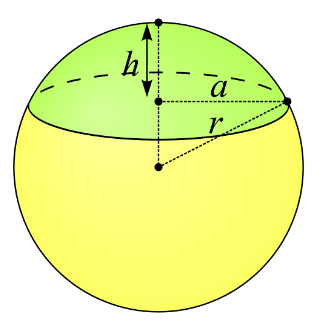# Spherical Cap Volume Formulas

The spherical cap is the portion of a sphere that lies above a plane of the sphere. If the base area, height and the sphere radius is known then the volume can be found out of the particular portion. Spherical dome is the term used synonymously to the spherical cap.

Sometimes in the question you will be given the base radius while in some you will be given as the sphere radius. IT important to differentiate and use the respective formula to find the surface area.The volume of the spherical cap with base radius is:

$\large V=\frac{\pi}{3}\,H^{2}(3R-H)$

Where,
H = height

### Solved example

Question: What is the surface of the spherical cap which has the height 40 cm and base radius as 30 cm?

Solution:

Given,
H = 30 cm
B = 40 cm

$$\begin{array}{l}V=\frac{\pi}{3}\,H^{2}(3R-H)\end{array}$$
$$\begin{array}{l}V=\frac{\pi}{3}\,40^{2}(3\times 30-40)\end{array}$$

=83809.52 cu.cm.